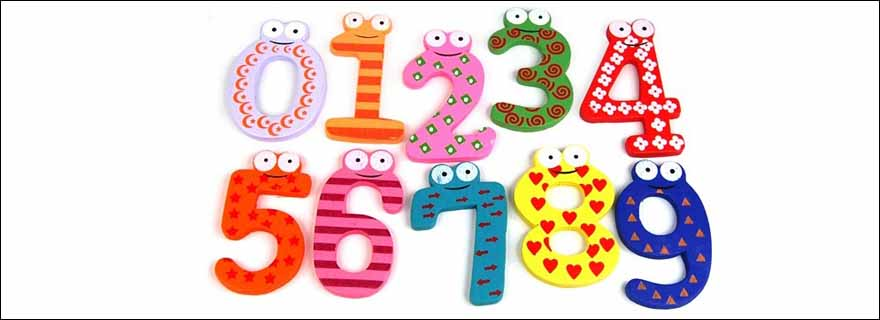# Name Number and its Significance

### Name Number and its SignificanceNumerology is a science of vibrations. Each alphabet has a distinct vibration represented by a number between 1 and 9. There are two systems of assignment of numbers to the alphabets of the English language. Numerologists use one or the other system and there is no rule as to which should be followed, it all depends on your choice. These systems are described here:

(1)The Pythagorean System …

This system came from the Greek mathematician Pythagoras who lived between 582 and 507 BC. Based on this system the English alphabet letters are assigned numbers as in the table below:

The Pythagorean System

English AlphabetsCorresponding Number
A,J,S1
B,K,T2
C,L,U3
D,M,V4
E,N,W5
F,O,X6
G,P,Y7
H,Q,Z8
I,R9

(2)The Chaldean System …

The origin of this system of numbers is not known exactly, however it is believed to be passed on to the Hebrews by the Chaldeans. The system has been applied to the English alphabet from the original ancient Chaldean and Hebrew alphabet. In this system no alphabet is assigned the number 9.

The Chaldean system

English AlphabetsCorresponding Number
A,I,J,Q,Y1
B,K,R2
C,G,L,S3
D,M,T4
E,H,N,X5
U,V,W6
O,Z7
F,P8

The Name Number …

Your name number must be compatible with your date of birth number. There is no way to change your date of birth number, but you can always modify your name in order to make its number compatible with your date of birth number.

So first of all you need to determine your date of birth number.

The Date of Birth Number …

Suppose you were born on 23rd of any month in any year then your date of birth number can be found by addition of both digits of this number till you get a corresponding single number and that will be your date of birth number. So working it out:

23=2+3=5

So your Date of Birth Number is Number 3

But if suppose your date of birth is 29 then you will have to carry on addition of the digits twice as follows:

29=2+9=11=1+1=2

so in this case your date of birth number will be 2. So it boils down to the fact that the date of birth number has to be a single number lying between 1 and 9

How to Find your Name Number …

It is your choice, which system as explained above, you follow. With experience you can see the results given by both systems.

Write down your name and then below it write the corresponding numbers from the above tables. Then add all the numbers and obtain a single number which will be your name number.

For example suppose your name is John Smith:

Note: for these examples we take the Pythagorean System

J+O+H+N+S+M+I+T+H
1+6+8+5+1+4+9+2+8=44=8

So your name number is Number 8

Let us consider another example for the name Ramesh Kumar:

R+A+M+E+S+H+K+U+M+A+R
9+1+4+5+1+8+2+3+4+1+9=47=4+7=11=1+1=2

So for the name Ramesh Kumar the name number is Number 2

Compatible Numbers …

Your Date of Birth NumberYour Name Number should be Either of these.
11,3,5,6
22,7
33,1,5,6
41,3,5,6,9
55,1,3,6
66,1,3,5
77,2
81,3,6,5,9
99,3,6,1,5

Note: In the above table the numbers are given in order of preference.

So you can see whether your name number needs modification or not. You can either rename yourself or add one or more alphabets strategically in order to modify the number.
Suppose your date of birth number is 8 then make sure that your name number corresponds to 1,3,6,5 or 9.

Just for example for the name KRISHAN=2+9+9+1+8+1+5=35=3+5=8.
The name number 8 needs to be changed to 1,3,6,5 or 9
So the easy way is to add another A or S such as KRISSHAN or KRISHAAN.
In either case the name number becomes 9.

Article by: Jyotirvid Pawan Kumar

What is Numerology